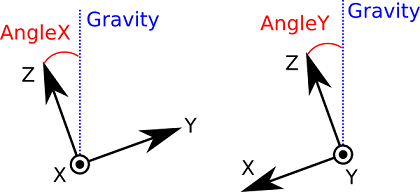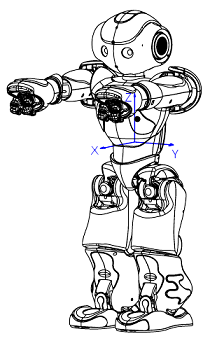Inertial unit¶

Specifications¶

The Inertial unit is made of:

• a 3-axis gyrometers with an angular speed of ~500°/s,
• a 3-axis accelerometer with an acceleration of ~2g.

Location¶

The Inertial unit is located in the torso with its own processor.

Position relative to the Torso frame.

Device X(m) Y(m) Z(m)
Accelerometer -0.008 0.00606 0.027
Gyrometer -0.008 0.006 0.029

Output data¶

The output data enables an estimation of the torso speed and attitude (Yaw, Pitch, Roll).

Compute Torso Angle Algorithm¶

An SoftBank Robotics algorithm computing the torso Angle from accelerometers and gyrometers is programming inside the inertial board.

This algorithm used the good proprieties of each sensor: accelerometer is the only absolute reference and give the good torso angle in static mode. When some motion is detected, the output angle is computed with gyrometers which have a good behavior in dynamic. However, integration of gyrometers creates a bias of the compute angle, so in dynamic mode, a fusion of compute angle from accelerometer and gyrometer is done to reduce this bias.Definition of AngleX and AngleY. [x, y, z] is the reference frame attached to torso.

Accessing value using ALMemory key name¶Glossary Site map Index Support Contact Legal Notices